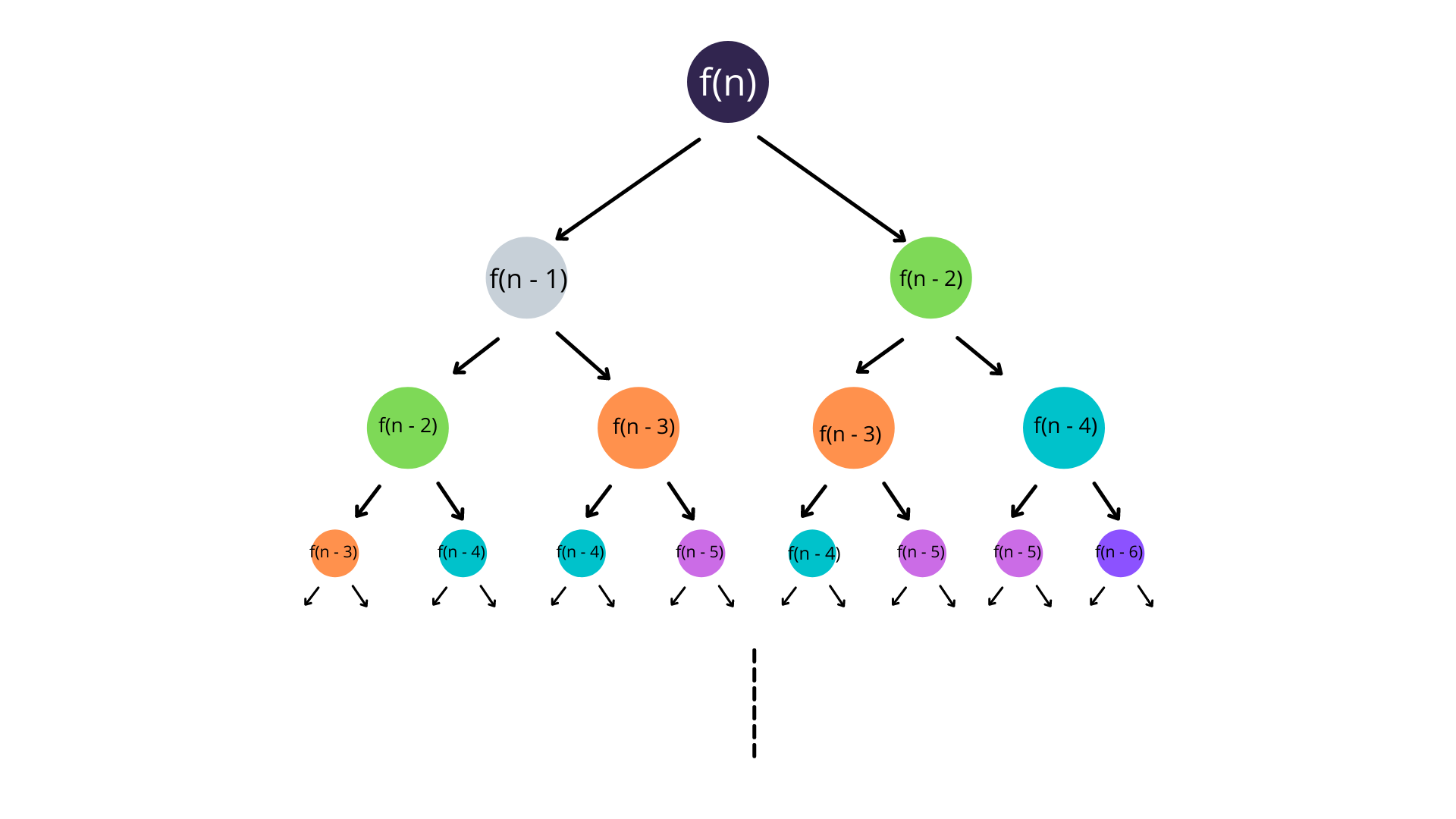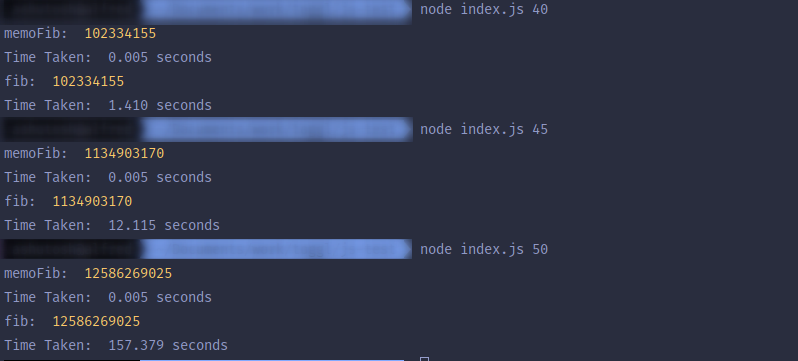# Memoization— the what, why and how?

#javascript#beginners#webdev#programming## What is Memoization?

Imagine, I'm your Math teacher and I gave you a problem to solve. You took 5 minutes to solve it, and the answer was correct. Good job!

10 Minutes later, I give you the exact same problem. What would you do?

You'll just tell me the same answer you did 10 minutes ago right away and spend the next 5 minutes talking to your crush, right? (unless you really love Math, haha)

Well, that right there is Memoization for you.

Memoization is caching expensive computations, so the computer doesn't have to do the same computation more than once, hence saving a lot of time and resources.

## Why do we need Memoization?

Memoization is most useful for common subset problems, where a smaller section of the problem has to be calculated multiple times to reach the final answer.

A good example of such problem is the Fibonacci series where the next number is the sum of the previous two.

``````0, 1, 1, 2, 3, 5, 8 ......
``````

this can be simplified using the following formula

`````` fib(n) = fib(n - 1) + fib(n - 2)
``````

As you can see, this equation can be written as a recursive function

``````
// return nth number from Fibonacci series
function fib(n) {
if (n === 0) {
return 0
}
if (n === 1) {
return 1
}
return fib(n - 1) + fib(n - 2)
}
``````

Now let's try our code: `node index.js <n>`Note: Code is running on a Ryzen 3400G with 16GB RAM

Well, this looks all good you might say.

Not so fast. Let's try with some bigger numbers.I guess, by now you can see what the problem is. The computation takes exponentially longer as we increase the number.

## How can Memoization help?

Before we solve the problem, let's see what the problem is.Looking at the above execution tree, we can see that problems are repeated more and more as we go down the tree.

So, the problem is we are doing the same computations multiple times.

The solution: Cache the computations or Memoize

Let's make the same `fib` a memoized function `memoFib`.

It's actually very simple to do so, we just need to introduce a cache.

``````
const cache = {}

function memoFib(n) {
if (cache[n]) return cache[n]
if (n === 0) {
return 0
}
if (n === 1) {
return 1
}
cache[n] = memoFib(n - 1) + memoFib(n - 2)
return cache[n]
}
``````

Time for the verdict:And we have a clear winner! The `memoFib` took almost constant time for all of these computations. While the `fib` went crazy.

Hence, it's evident how important Memoization is.

## How can I Memoize?

Well, if you have been writing code for a while you must have done it in one way or the other.

The simplest way to do it is to cache function calls using a dictionary-like structure e.g. a Map or an Object in JavaScript.

If you're a React developer, you might have come across hooks like `useMemo` or `useCallback`. Both of these hooks are an implementation of Memoization.

These hooks Memoize the returned value, so the value is not computed on every render of the React Component. Hence, making your apps faster.Return to All Articles